The UNIVARIATE Procedure
 Calculating Percentiles

The UNIVARIATE procedure automatically computes the 1st, 5th, 10th, 25th, 50th, 75th, 90th, 95th, and 99th percentiles (quantiles), as well as the minimum and maximum of each analysis variable. To compute percentiles other than these default percentiles, use the PCTLPTS= and PCTLPRE= options in the OUTPUT statement.

You can specify one of five definitions for computing the percentiles with the PCTLDEF= option. Letbe the number of nonmissing values for a variable, and let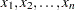represent the ordered values of the variable. Let theth percentile be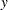, set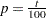, and let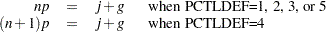whereis the integer part of np, andis the fractional part of np. Then the PCTLDEF= option defines theth percentile,, as described in the following table.

PCTLDEF

Description

Formula

1

weighted average at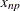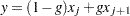where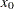is taken to be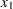2

observation numbered closest to np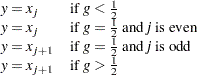3

empirical distribution function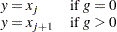4

weighted average aimedat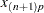where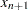is taken to be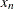5

empirical distribution function with averaging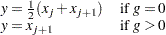Weighted Percentiles

When you use a WEIGHT statement, the percentiles are computed differently. The 100th weighted percentileis computed from the empirical distribution function with averaging: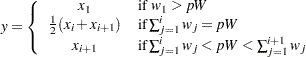where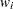is the weight associated with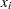and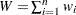is the sum of the weights.

Note that the PCTLDEF= option is not applicable when a WEIGHT statement is used. However, in this case, if all the weights are identical, the weighted percentiles are the same as the percentiles that would be computed without a WEIGHT statement and with PCTLDEF=5.

Confidence Limits for Percentiles

You can use the CIPCTLNORMAL option to request confidence limits for percentiles, assuming the data are normally distributed. These limits are described in Section 4.4.1 of Hahn and Meeker (1991). When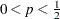, the two-sided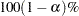confidence limits for the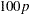th percentile are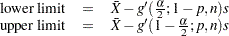whereis the sample size. When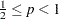, the two-sidedconfidence limits for theth percentile are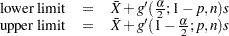One-sidedconfidence bounds are computed by replacing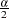byin the appropriate preceding equation. The factor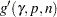is related to the noncentraldistribution and is described in Owen and Hua (1977) and Odeh and Owen (1980). See Example 4.10.

You can use the CIPCTLDF option to request distribution-free confidence limits for percentiles. In particular, it is not necessary to assume that the data are normally distributed. These limits are described in Section 5.2 of Hahn and Meeker (1991). The two-sidedconfidence limits for theth percentile are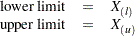where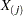is theth order statistic when the data values are arranged in increasing order: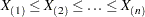The lower rankand upper rankare integers that are symmetric (or nearly symmetric) around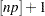, where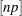is the integer part of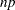andis the sample size. Furthermore,andare chosen so that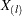and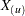are as close to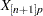as possible while satisfying the coverage probability requirement,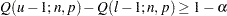where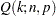is the cumulative binomial probability,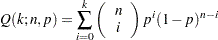In some cases, the coverage requirement cannot be met, particularly whenis small andis near 0 or 1. To relax the requirement of symmetry, you can specify CIPCTLDF(TYPE = ASYMMETRIC). This option requests symmetric limits when the coverage requirement can be met, and asymmetric limits otherwise.

If you specify CIPCTLDF(TYPE = LOWER), a one-sidedlower confidence bound is computed as, whereis the largest integer that satisfies the inequality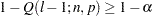with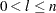. Likewise, if you specify CIPCTLDF(TYPE = UPPER), a one-sidedlower confidence bound is computed as, whereis the largest integer that satisfies the inequality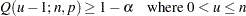Note that confidence limits for percentiles are not computed when a WEIGHT statement is specified. See Example 4.10.Previous Page | Next Page | Top of Page